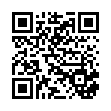# Statistical Methods Review Notes Ch1 to 3.4 .pdf

### File information

Author: Jonathan

This PDF 1.5 document has been generated by MicrosoftÂ® Word 2013, and has been sent on pdf-archive.com on 30/01/2016 at 01:16, from IP address 97.103.x.x. The current document download page has been viewed 1024 times.
File size: 344.75 KB (6 pages).
Privacy: public file

### Document preview

STASTISTICAL THINKING
Word Bank (use your textbook to define these terms):
A. unit B. population C. sample D. variable E. qualitative variable F. quantitative variable
G. dataset H. statistic I. parameter J. descriptive statistic k. inferential statistic
___ 1. Collection of values of all variables associated with a unit, often displayed as an array.
___ 2. An observed property of a unit in a population.
___ 3. Estimation, prediction, or hypothesis test about some unknown characteristic of a
population based on randomly sampled data.
___ 4. A numerical value obtained by measuring some characteristic.
___ 5. An object about which we collect data.
___ 6. A classification of some characteristic based on discrete categories.
___ 7. A characterizing attribute of a sample.
___ 8. A subset of the units of a population.
___ 9. A set of units that we are interested in studying.
___ 10. A numerical value summarizing some attribute of a sample, such as the class relative
frequency, measures of central tendency, or measures of variability.
___ 11. A characterizing attribute of a population, often unknown.

An example of statistical thinking:
Does a massage enable the muscles of a tired athletes to recover from exertion faster than
usual? To answer this question, researchers recruited eight amateur boxers to participate in an
experiment. After a 10-minute workout in which each boxer threw 400 punches, half of the
boxers were given a 20-minute massage and the other half rested for 20 minutes. Before they
returned to the ring for a second workout, the heart rate (bpm) and blood lactate level
(micromoles) were recorded for each boxer. The researchers found no difference in the means
of the two groups of boxers for either variable.
a. Identify the data collection method.
b. Identify the experimental units of the study.
c. Identify the variables measured and their type.
d. What is the inference drawn from the analysis?
e. Comment on whether this inference can be made of all athletes.

METHODS FOR DESCRIBING SETS OF DATA
Graphical methods for representing data:
Quantitative data:

Dot-plot
Stem-n-leaf display
Histogram

Qualitative data:

Bar graph
Pareto Diagram
Pie chart

Measures of central tendency:

Mean ( : the sum of all values, xi, divided by the number of values, n.
Median (M): the middle value of the sorted array of measurements.
o n is odd  M is the middle index
o n is even  M is the average of the two middle indices
Mode: the measurement(s) that occur most frequent in within the dataset.
o e.g.: 3, 3, 4, 4  no mode
o e.g.: 3, 3, 3, 4, 4  mode is 3
o e.g.: 3, 3, 4, 4, 5  mode is 3 and 4

Detecting skewness by comparing the mean and median:
Rule of thumb: Look at the tail’s direction.

if M &lt;  right skewed
if &lt; M  right skewed
if = M  symmetric

Measures of variability:

Range (R): xMAX – xMIN
Variance (s2): ∑(xi – )2/(n-1)
Standard deviation (s): √(s2)
Coefficient of Variance (CV): s/| |
o Note: used as an independent measure of variance to compare the variability of
differing units.

Measures of relative position:

Percentile scores (ith percentile): divides an ordered data set into 100 equal parts.
Quartile scores (Q1, Q2, Q3): divides an ordered data set into 4 equal parts.
o Note: Q2 = M
z-scores: a standardized score of some value, x, relative its position on the standard
normal curve.
o z(x) = (x – μ)/σ
o Note: for a standard, normalized curve, μ = 0 and σ = 1.
o Rule of thumb: |z| &gt; 3  outlier
Five number summary:
o xMIN, Q1, M, Q3, xMAX
o Note: Interquartile Range (IQR) = Q3 – Q1
 x &lt; Q1 – 3(IQR) OR x &gt; Q3 + 3(IQR)  outlier

Practice:
Find the mean, variance, and standard deviation of the dataset:

x
1
2
3
4
5
∑’s

freq(x) midpoint x∙f x2∙f
5
9
7
3
1
25
-

= ∑(x∙f)/∑(f)
s = [[∑(x2∙f) – [∑(x∙f)2/∑(f)]] / ∑(f) – 1
2

Chebyshev’s Rule:
For any dataset, regardless of the shape of its frequency distribution, at least [1 – (1/k2)] of the
measurements will fall within k standard deviations of the mean, where k is an integer greater
than 1.
Empirical Rule:
For any dataset that is normally distributed:

Approximately 68% of the measurements fall within 1 standard deviation of the mean
Approximately 95% of the measurements fall within 2 standard deviations of the mean
Approximately 99.7% of the measurements fall w/in 3 standard deviations of the mean

Practice:
1. A dataset has a mean of 13 and a standard deviation of 3. What percentage of the data
falls between 10 and 16? What percentage falls between 7 and 13? What percentage
falls between 7 and 16? (HINT: Do we know the shape?).

2. A dataset with a bell-shaped frequency distribution has a mean of 110 and standard
deviation of 15. What percentage of the data falls between 95 and 125?

PROBABILITY
Formula Bank:
A. P(A) + P(B) – P(A∩B)

B. P(A) ∙ P(B)

C. P(A∩B) = 0

D. P(A) + P(B)

E. AC = 1 – A

___ 1. The probability of event A not occurring.
___ 2. The probability of the union of two events.
___ 3. The probability of the intersection of two mutually exclusive events.
___ 4. The probability of an event occurring OR some other event occurring, where both events
are independent of each other.
___ 5. The probability an event AND some other event occurring, where both events are
independent of each other.

PRACTICE:
1. Given:
A = {P(E1), P(E2), P(E3), P(E5), P(E6)}
B = {P(E2), P(E3), P(E4), P(E7)}
P(E2) = P(E3) = 1/5
P(E4) = P(E5) = 1/20
P(E6) = 1/10
P(E7) = 1/5
(HINT: Use this information to draw a Venn diagram)
a. Find P(A)
b. Find P(BC)
c. Find P(AC∩B)

2. The effect of guilt emotion on how a decision maker focuses on a problem was
investigated. A total of 171 volunteer students were each randomly assigned to one of
Immediately after the task, the students were presented with a decision problem where
the stated option has predominantly negative features. The results are summarized as
follows:
Emotional State
Guilt
Anger
Neutral
TOTALS

Choose Option
45
8
7
60

Do Not Choose Op.
12
50
49
111

TOTALS
57
58
56
171

Suppose one of the participants is selected at random. Find the probability that the
respondent is:
a. assigned to the guilty state
b. chooses the stated option
c. assigned to the guilty state AND chooses the stated option
d. assigned to the guilty state OR chooses the stated option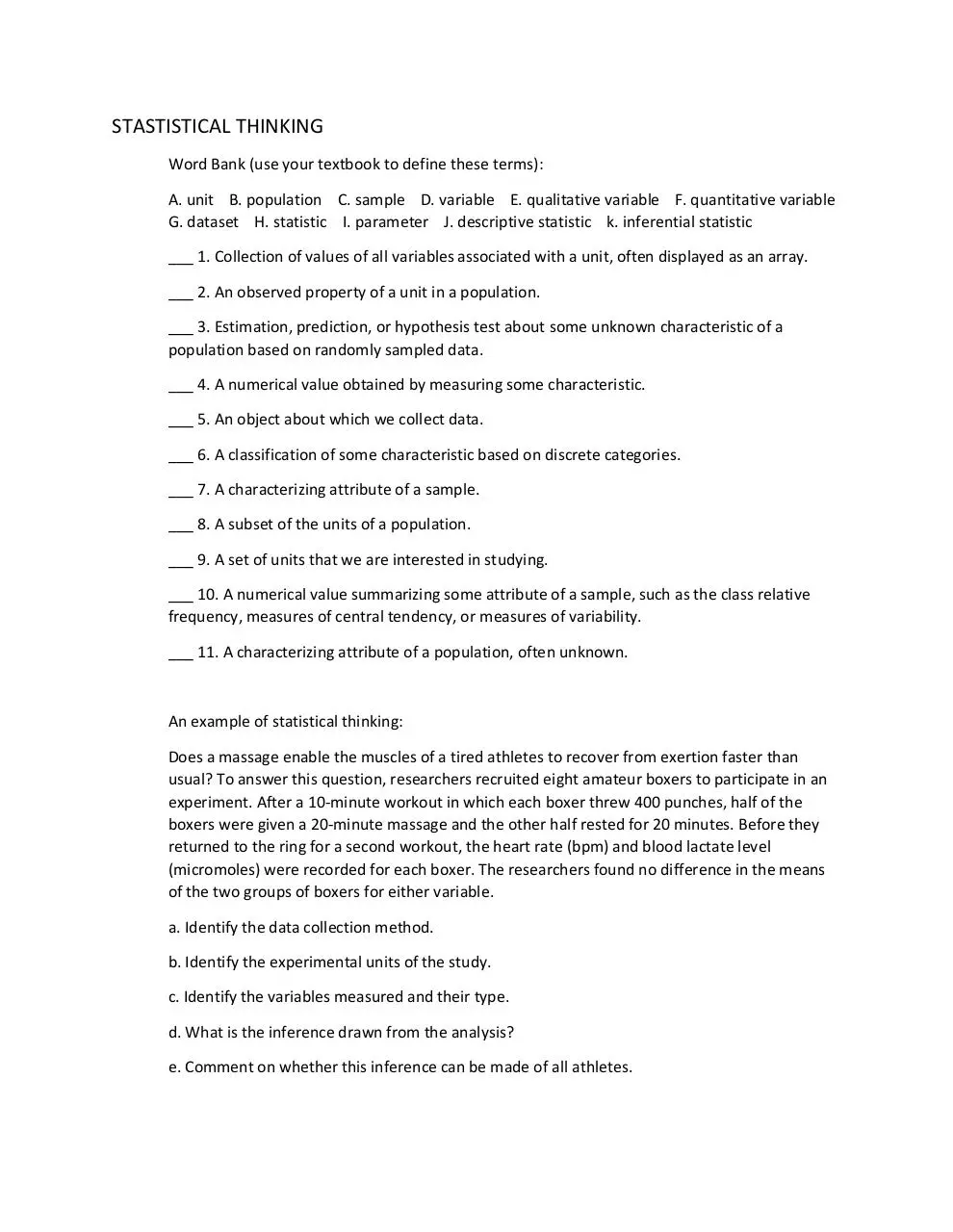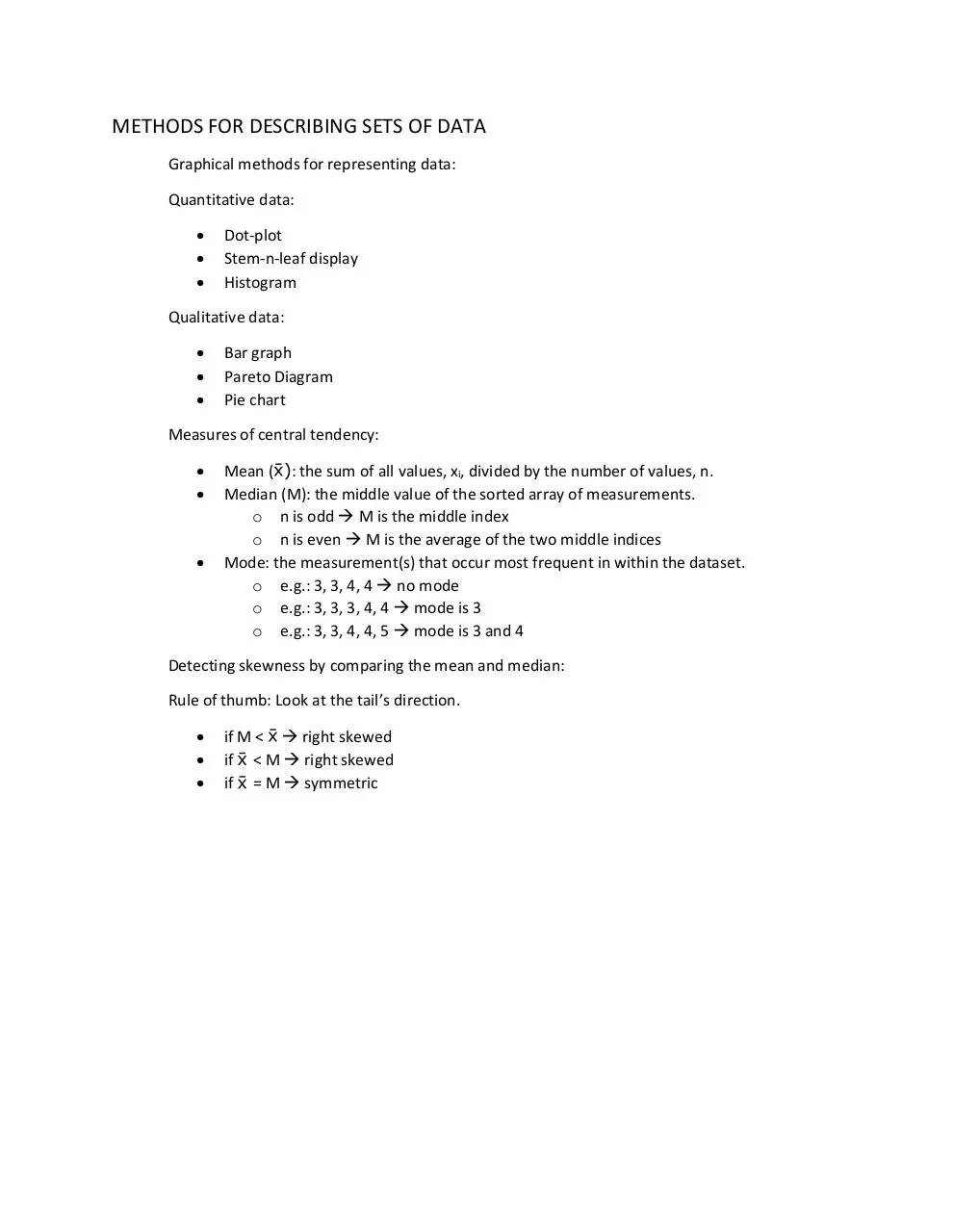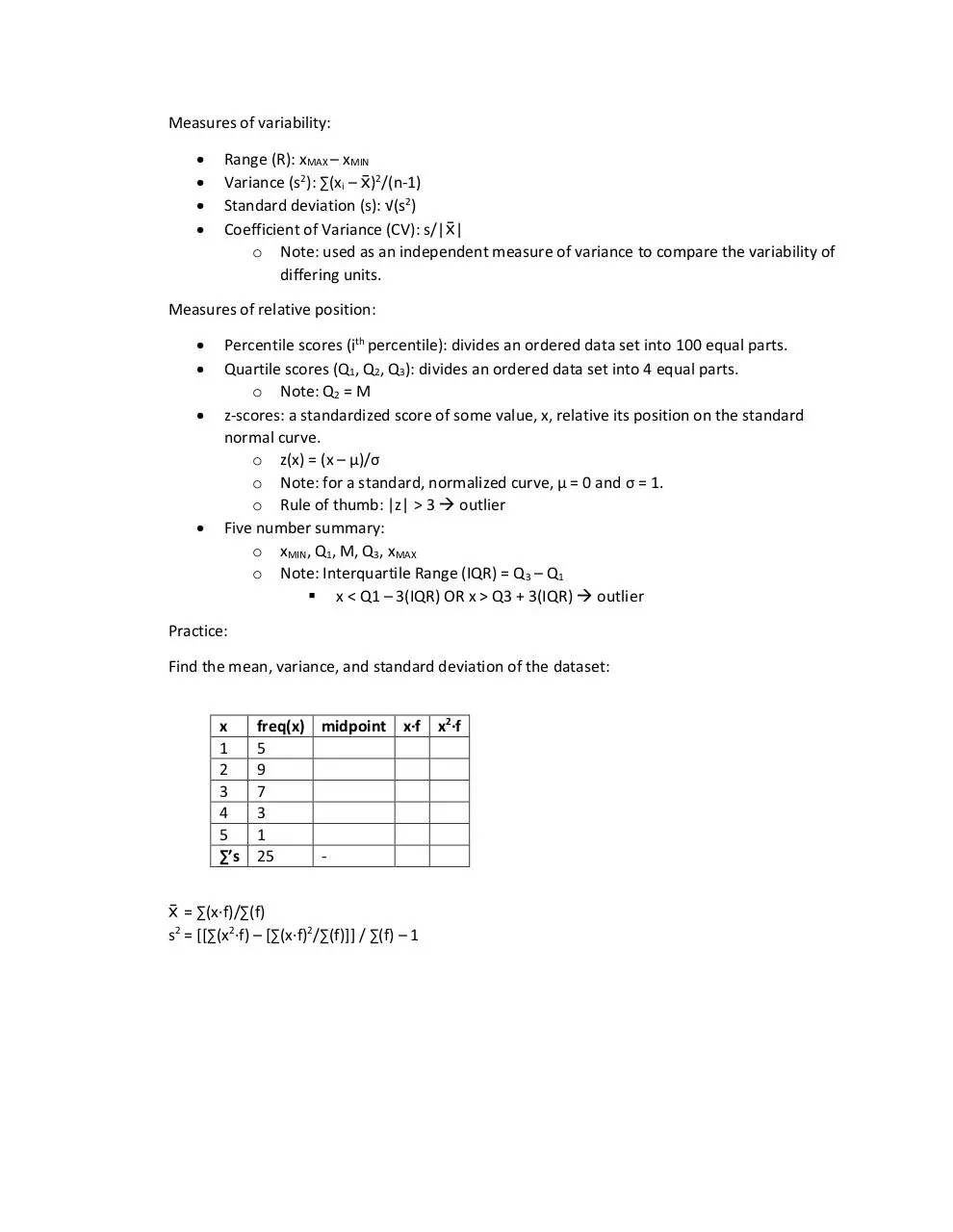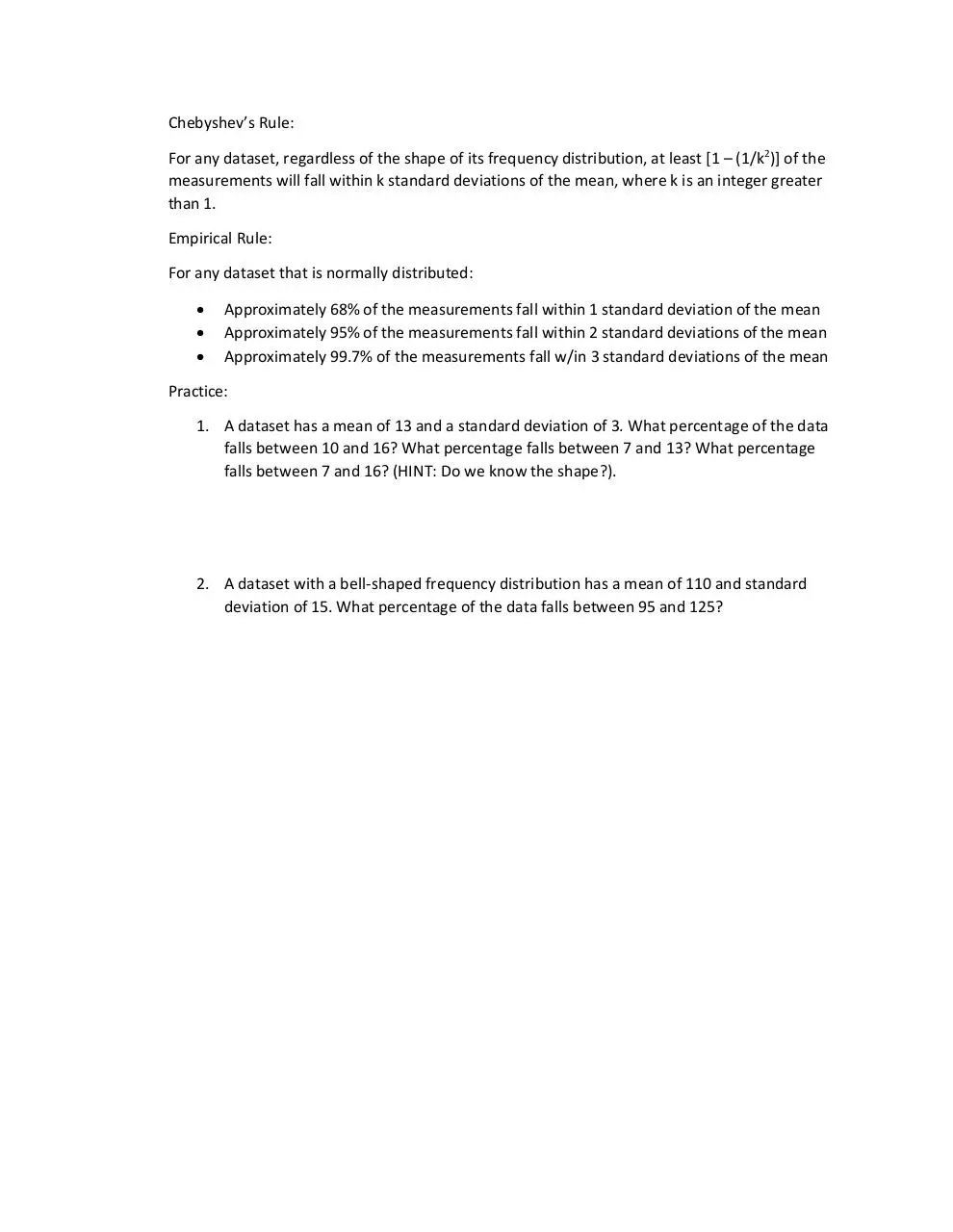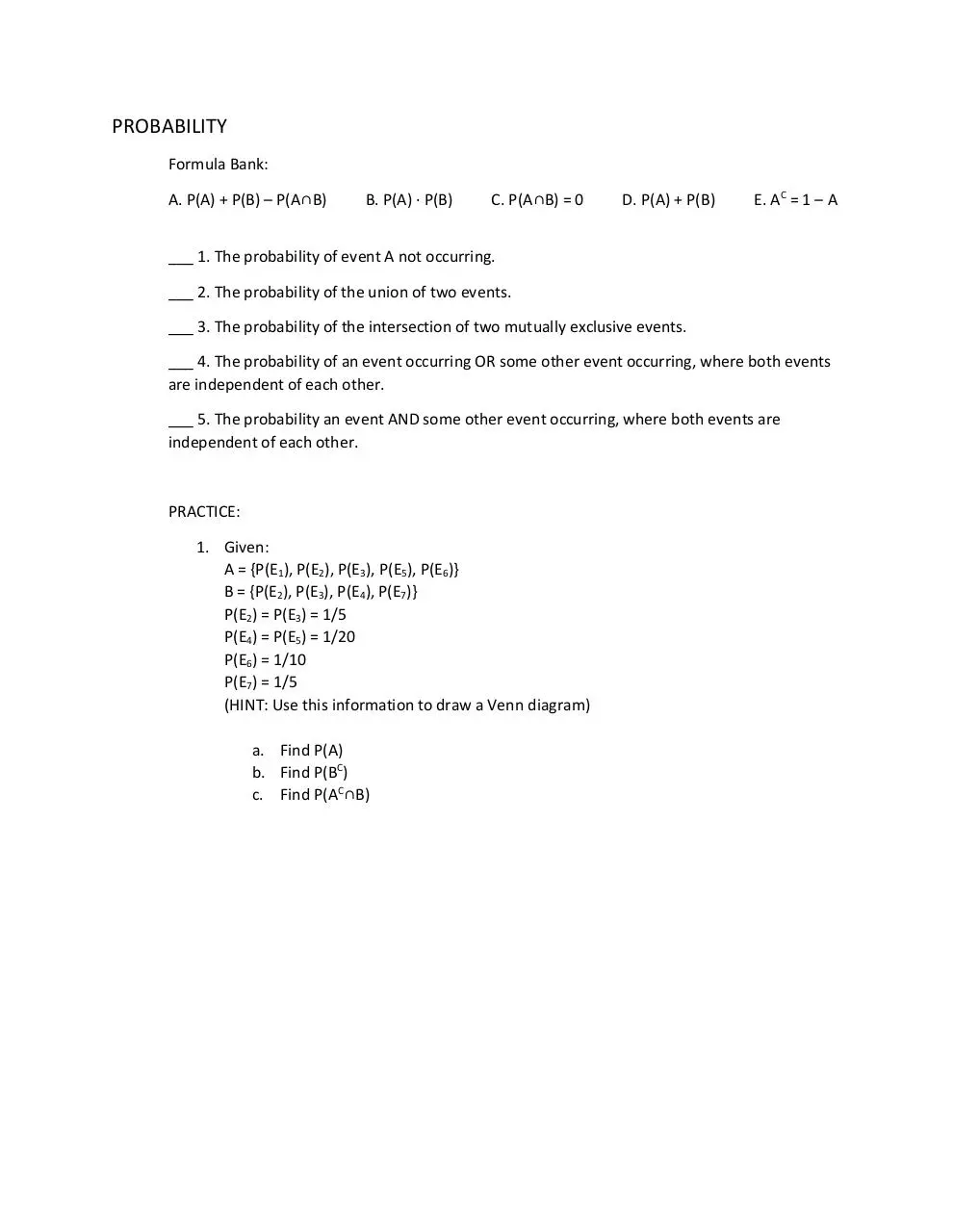Statistical Methods - Review Notes - Ch1 to 3.4.pdf (PDF, 344.75 KB)

### Share on social networks

#### HTML Code

Copy the following HTML code to share your document on a Website or Blog